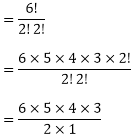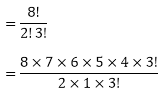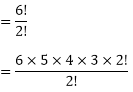×#### Thank you for registering.

One of our academic counsellors will contact you within 1 working day.

Click to Chat

1800-1023-196

+91-120-4616500

CART 0

• 0

MY CART (5)

Use Coupon: CART20 and get 20% off on all online Study Material

ITEM
DETAILS
MRP
DISCOUNT
FINAL PRICE
Total Price: Rs.

There are no items in this cart.
Continue Shopping• Complete JEE Main/Advanced Course and Test Series
• OFFERED PRICE: Rs. 15,900
• View Details

```Chapter 16: Permutations – Exercise 16.5

Permutations – Exercise – 16.5 – Q.1(i)

There are 12 letters in the word 'INDEPENDENCE' out of which 2 are D'S, 3 are N'S, 4 are E'S and the rest are all distinct.

so, the total number of words= 11 × 10 × 9 × 8 × 7 × 6 × 5 = 1663200.

Permutations – Exercise – 16.5 – Q.1(ii)

There are 12 letters in the word 'INTERMEDIATE' out of which 2 are I'S, 2 are T'S, 3 are E'S and the rest are all distinct.

so, the total number of words= 11 × 10 × 9 × 8 × 6 × 5 × 4 × 3

= 19958400

Permutations – Exercise – 16.5 – Q.1(iii)

There are 7 letters in the word 'ARRANGE' out of which 2 are A'S, 2 are R'S, and the rest are all distinct.

So, the total number of words= 7 × 6 × 5 × 2 × 3

= 1260

Permutations – Exercise – 16.5 – Q.1(iv)

There are 5 letters in the word 'INDIA' out of which 2 are I'S, and the rest are all distinct.

so, the total number of= 60

Permutations – Exercise – 16.5 – Q.1(v)

There are 8 letters in the word 'PAKISTAN' out of which 2 are A'S, and the rest are all distinct.

So, the total number of words= 8 × 7 × 6 × 5 × 4 × 3

= 20160

Permutations – Exercise – 16.5 – Q.1(vi)

There are 6 letters in the word 'RUSSIA' out of which 2 are S's, and the rest are all distinct.

So, the total number of words= 6 × 5 × 4 × 3

= 360

Permutations – Exercise – 16.5 – Q.1(vii)

There are 6 letters in the word 'SERIES' out of which 2 are S's, 2 are E's and the rest are all distinct.

so, the total number of words= 6 × 5 × 2 × 3

= 180

Permutations – Exercise – 16.5 – Q.1(viii)

There are 9 letters in the word 'EXERCISES' out of which 3 are E's, 2 are S's and the rest are all distinct.

So, the total number of words= 9 × 8 × 7 × 6 × 5 × 2

= 30240

Permutations – Exercise – 16.5 – Q.1(ix)

There are 14 letters in the word 'CONSTANTINOPLE' out of which 2 are O's, 3 are N's, 2 are T's and the rest are all distinct.

So, the total number ofPermutations – Exercise – 16.5 – Q.2

There are 4 consonants in the word 'ALGEBRA'.

The number of ways to arrange these consonants = 4!

There are 3 vowels in the given word of which 2 are A's

The vowels can be arranged among themselves in 3!/2! ways.

Hence, the required number of arrangements= 72

Permutations – Exercise – 16.5 – Q.3

In the word 'UNIVERSITY' there are 10 letters of which 2 are I's.

There are 4 vowels in the given word of which 2 are I's.

These vowels can be put together in 4!/2! ways.

Considering these 4 vowels as one letter there are 7 letters which can be arranged in 7! ways.

Hence, by fundamental principle of multiplication, the required number of arrangements is= 60480.

Permutations – Exercise – 16.5 – Q.4

There are 3a's, 2b's and 4c's.

So, the number of arrangements 9!= 9 × 4 × 7 × 5

= 1260.

Hence, the total number of arrangements are 1260.

Permutations – Exercise – 16.5 – Q.5

There are 8 letters in the word 'PARALLEL' out of which A's and 3 are L's and the rest are all distinct.

So, total number of words= 8 × 7 × 6 × 5 × 2

= 3360

Considering all L's together and treating them as one letter we have 6 letters out of which A repeats 2 times and others are distinct. These 6 letters can be arranged in 6!/2! ways.

So, the number of words in which all L's come together= 6 × 5 × 4 × 3 = 360

Hence, the number of words in which all L's do not come together

= 3360 - 360

= 3000.

Permutations – Exercise – 16.5 – Q.6

There are 6 letters in the word 'MUMBAI' out of which 2 are M's and the rest are all distinct.

Considering both M's together and treating as one letter we have 5 letters. These 5 letters can be arranged in 5! ways.

Hence, the total number of arrangement = 5!

= 5 × 4 × 3 × 2 × 1

= 120

Permutations – Exercise – 16.5 – Q.7

Total number of digits are = 7

There are 4 odd digits 1, 1, 3, 3 and 4 odd places (1, 3, 5, 7)

So, odd digits can be arranged in odd places in 4!/2!2! ways

The remaining 3 even digits 2, 2, 4 can be arranged in 3 even places in 3!/2! ways.

Hence, the total number of Numbers```### Course Features

• 728 Video Lectures
• Revision Notes
• Previous Year Papers
• Mind Map
• Study Planner
• NCERT Solutions
• Discussion Forum
• Test paper with Video Solution